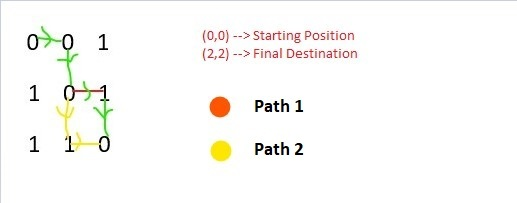# Maze With N doors and 1 Key

Given an N * N binary maze where a 0 denotes that the position can be visited and a 1 denotes that the position cannot be visited without a key, the task is to find whether it is possible to visit the bottom-right cell from the top-left cell with only one key along the way. If possible then print “Yes” else print “No”.
Example:

Input: maze[][] = {
{0, 0, 1},
{1, 0, 1},
{1, 1, 0}}
Output: YesApproach: This problem can be solved using recursion, for very possible move, if the current cell is 0 then without altering the status of the key check whether it is the destination else move forward. If the current cell is 1 then the key must be used, now for the further moves the key will be set to false i.e. it’ll never be used again on the same path. If any path reaches the destination then print Yes else print No.

Below is the implementation of the above approach:

## C++

 `// C++ implementation of the approach ` `#include ` `using` `namespace` `std;`   `// Recursive function to check whether there is  ` `// a path from the top left cell to the  ` `// bottom right cell of the maze ` `bool` `findPath(vector> maze, ` `              ``int` `xpos, ``int` `ypos, ``bool` `key)` `{` `    `  `    ``// Check whether the current cell is` `    ``// within the maze` `    ``if` `(xpos < 0 || xpos >= maze.size() || ` `        ``ypos < 0 || ypos >= maze.size())` `        ``return` `false``;`   `    ``// If key is required to move further` `    ``if` `(maze[xpos][ypos] == ``'1'``)` `    ``{` `        `  `        ``// If the key hasn't been used before` `        ``if` `(key == ``true``)`   `            ``// If current cell is the destination` `            ``if` `(xpos == maze.size() - 1 && ` `                ``ypos == maze.size() - 1)` `                ``return` `true``;`   `        ``// Either go down or right` `        ``return` `findPath(maze, xpos + 1,` `                        ``ypos, ``false``) ||` `               ``findPath(maze, xpos, ` `                        ``ypos + 1, ``false``);`   `        ``// Key has been used before` `        ``return` `false``;` `    ``}` `    `  `    ``// If current cell is the destination` `    ``if` `(xpos == maze.size() - 1 &&` `        ``ypos == maze.size() - 1)` `        ``return` `true``;`   `    ``// Either go down or right` `    ``return` `findPath(maze, xpos + 1,` `                    ``ypos, key) ||` `           ``findPath(maze, xpos, ` `                    ``ypos + 1, key);` `}`   `bool` `mazeProb(vector> maze,` `              ``int` `xpos, ``int` `ypos)` `{` `    ``bool` `key = ``true``;` `    ``if` `(findPath(maze, xpos, ypos, key))` `        ``return` `true``;` `        `  `    ``return` `false``;` `}`   `// Driver code` `int` `main()` `{` `    ``vector> maze = { { ``'0'``, ``'0'``, ``'1'` `},` `                                 ``{ ``'1'``, ``'0'``, ``'1'` `},` `                                 ``{ ``'1'``, ``'1'``, ``'0'` `} };` `    ``int` `n = maze.size();`   `    ``// If there is a path from the cell (0, 0)` `    ``if` `(mazeProb(maze, 0, 0))` `        ``cout << ``"Yes"``;` `    ``else` `        ``cout << ``"No"``;` `}`   `// This code is contributed by grand_master`

## Python3

 `# Python3 implementation of the approach`   `# Recursive function to check whether there is ` `# a path from the top left cell to the ` `# bottom right cell of the maze` `def` `findPath(maze, xpos, ypos, key):`   `    ``# Check whether the current cell is ` `    ``# within the maze` `    ``if` `xpos < ``0` `or` `xpos >``=` `len``(maze) ``or` `ypos < ``0` `\` `                            ``or` `ypos >``=` `len``(maze):` `        ``return` `False`   `    ``# If key is required to move further` `    ``if` `maze[xpos][ypos] ``=``=` `'1'``:`   `        ``# If the key hasn't been used before` `        ``if` `key ``=``=` `True``:`   `            ``# If current cell is the destination` `            ``if` `xpos ``=``=` `len``(maze)``-``1` `and` `ypos ``=``=` `len``(maze)``-``1``:` `                ``return` `True`   `            ``# Either go down or right` `            ``return` `findPath(maze, xpos ``+` `1``, ypos, ``False``) ``or` `\` `            ``findPath(maze, xpos, ypos ``+` `1``, ``False``)`   `        ``# Key has been used before` `        ``return` `False`   `    ``# If current cell is the destination` `    ``if` `xpos ``=``=` `len``(maze)``-``1` `and` `ypos ``=``=` `len``(maze)``-``1``:` `        ``return` `True`   `    ``# Either go down or right` `    ``return` `findPath(maze, xpos ``+` `1``, ypos, key) ``or` `\` `           ``findPath(maze, xpos, ypos ``+` `1``, key)`     `def` `mazeProb(maze, xpos, ypos):` `    ``key ``=` `True` `    ``if` `findPath(maze, xpos, ypos, key):` `        ``return` `True` `    ``return` `False`   `# Driver code ` `if` `__name__ ``=``=` `"__main__"``:`   `    ``maze ``=` `[[``'0'``, ``'0'``, ``'1'``], ` `            ``[``'1'``, ``'0'``, ``'1'``], ` `            ``[``'1'``, ``'1'``, ``'0'``]]` `    ``n ``=` `len``(maze)` `    `  `    ``# If there is a path from the cell (0, 0)` `    ``if` `mazeProb(maze, ``0``, ``0``):` `        ``print``(``"Yes"``)` `    ``else``:` `        ``print``(``"No"``)`

Output:

```Yes

```

Time Complexity: O(2N)

Attention reader! Don’t stop learning now. Get hold of all the important DSA concepts with the DSA Self Paced Course at a student-friendly price and become industry ready.

My Personal Notes arrow_drop_upCheck out this Author's contributed articles.

If you like GeeksforGeeks and would like to contribute, you can also write an article using contribute.geeksforgeeks.org or mail your article to contribute@geeksforgeeks.org. See your article appearing on the GeeksforGeeks main page and help other Geeks.

Please Improve this article if you find anything incorrect by clicking on the "Improve Article" button below.

Improved By : Akanksha_Rai, grand_master